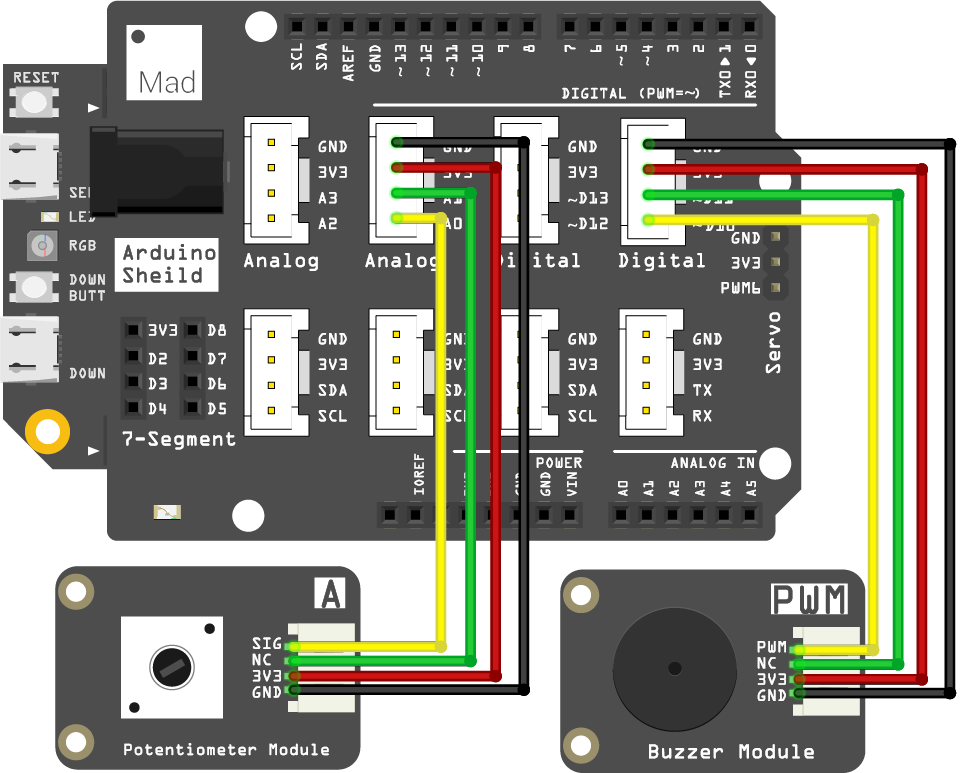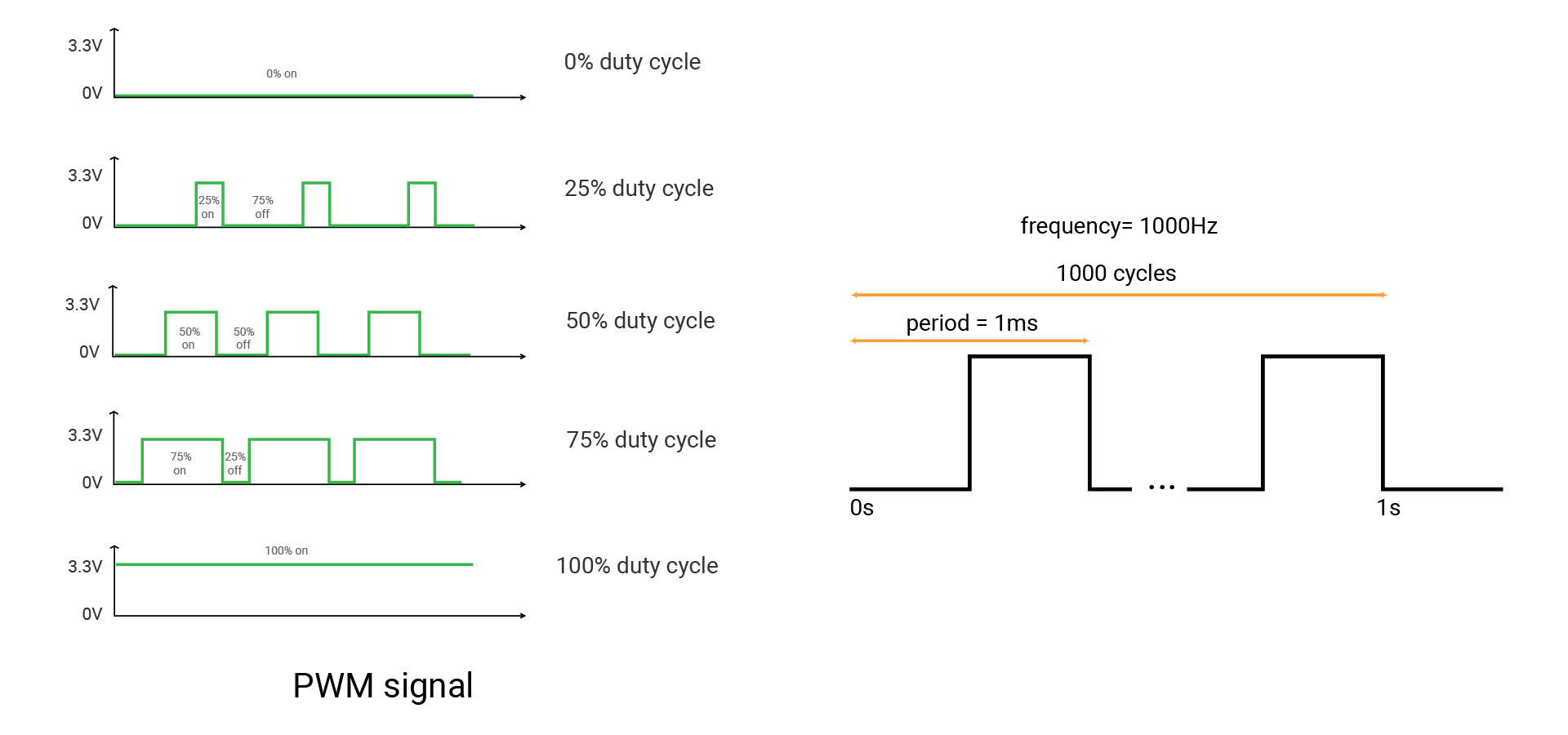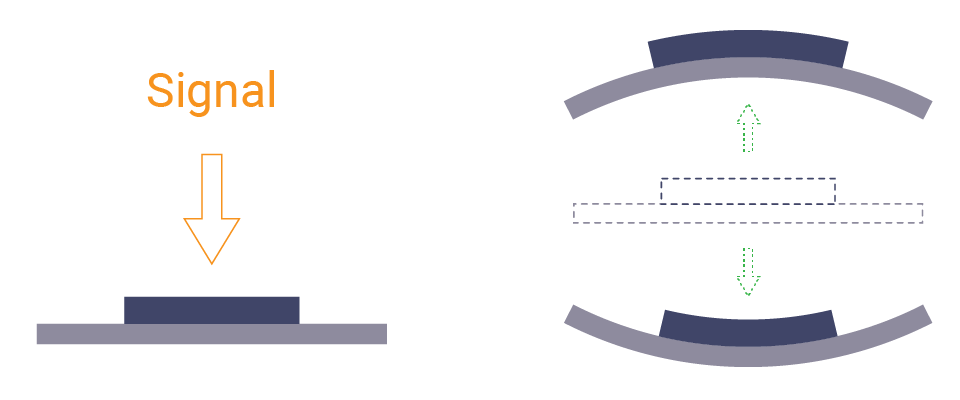# Mission5_Buzzer

In this project, you will get a buzzer buzzing. And its sound will change as you turn the potentiometer.

## What you need​

The parts you will need are all included in the Maker kit.

• SwiftIO board
• Shield
• Buzzer module
• Potentiometer module
• 4-pin cable

## Circuit​

1. Place the shield on top of your SwiftIO board.

2. Connect the potentiometer module to pin A0 using a 4-pin cable.

3. Connect the buzzer module to pin PWM2B (D10).## Example code​

``// Import the SwiftIO library to use everything in it.import SwiftIO// Import the board library to use the Id of the specific board.import MadBoard// Initialize the analog pin for the potentiometer.let a0 = AnalogIn(Id.A0)// Initialize the PWM output pin.let buzzer = PWMOut(Id.PWM2B)while true {    // Read the input voltage.    let value = a0.readPercent()        // calculate the float value into an integer to serve as frequency.    let frequency = Int(400 + 2000 * value)     // Set PWM parameters.    buzzer.set(frequency: frequency, dutycycle: 0.5)     // Set the duration of the notes.    sleep(ms: 20) }``

## Background​

### Pulse Width Modulation (PWM)​

Pulse Width Modulation (PWM) can simulate analog results digitally. The signal is still a square wave that switches between on and off. The duration of the "on-time" is called the pulse width. So this technique will change the duration of the high level relative to the low level and switch extremely quickly between them. In this way, it will simulate the voltage between fully open (3.3 volts) and off (0 volts).

In this case, if you repeat this switching pattern with LEDs fast enough, your eyes cannot notice the change and the signal seems to be a stable voltage between 0 and 3.3v. Thus the LED would show different brightness to your eyes.

Now come more concepts. A fixed time period consists of on and off time. The period is the inverse of the PWM frequency. For example, when the PWM frequency is 1000 Hz, one period is 1 millisecond.

The duty cycle is the percentage of on-time of output signal during one period. Its range is 0-1. 1 means the output is always on. 0 means the voltage is always low. And the signal with a 0.5 duty cycle is on for 50% of the time and off for 50% of the time.### Buzzer​

The buzzer can buzz when you apply voltages. There is a diaphragm inside it. It can move back and forth as the signal switches between on and off. The vibration causes the surrounding air to vibrate. Thus the buzzer produces the sound.There are two kinds of buzzers.

• An active buzzer has an internal circuit to create alternating voltage. So you can connect it to power. But it can only generate a sound since the voltage cannot change.
• A passive buzzer needs PWM signals. When the signal switches between on and off, the internal diaphragm moves. The frequency of the signal will influence the pitch. A higher frequency will produce a higher pitch. That's how this projects works.

## Code analysis​

``import SwiftIOimport MadBoard``

First, import the two libraries: SwiftIO and SwiftIOBoard to use everything in it. SwiftIO is used to control the input and output of the SwiftIO board. SwiftIOBoard defines the pin name of the board.

``let a0 = AnalogIn(Id.A0)let buzzer = PWMOut(Id.PWM2B)``

Initialize the analog pin (A0) for the potentiometer and the PWM pin (PWM2B) for the buzzer.

``while true {    let value = a0.readPercent()    let frequency = Int(400 + 2000 * value)     buzzer.set(frequency: frequency, dutycycle: 0.5)    sleep(ms: 20) }``

The potentiometer will control the buzzer all the time, so you write the code in the loop `while true`.

In the loop,

1. read the analog value and store it in the constant `value`.
2. set the PWM signal. You will need `set(frequency:dutycycle:)`. This method has two parameters: frequency and duty cycle. Its frequency is related to the analog value above. While the value is too small to be used as a frequency, so you do some calculations. The duty cycle doesn't really matter in this project, and you can set it to 0.5 here or any value.
3. Use the function `sleep(ms:)` to make each pitch will last about 20ms.

## Reference​

PWMOut - set the PWM signal.